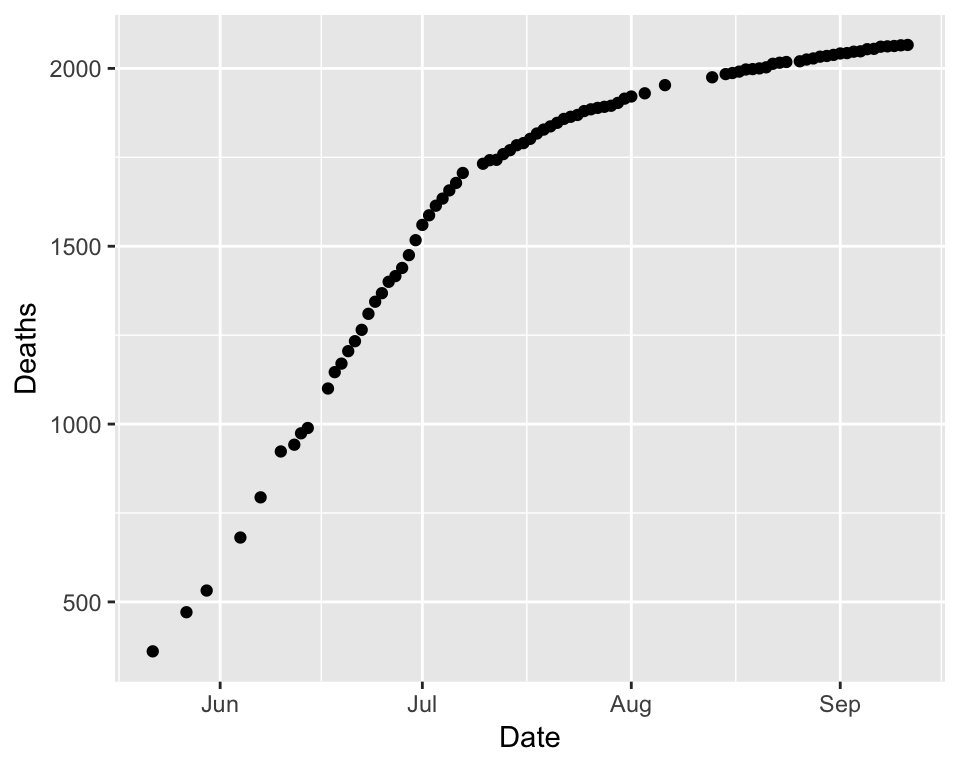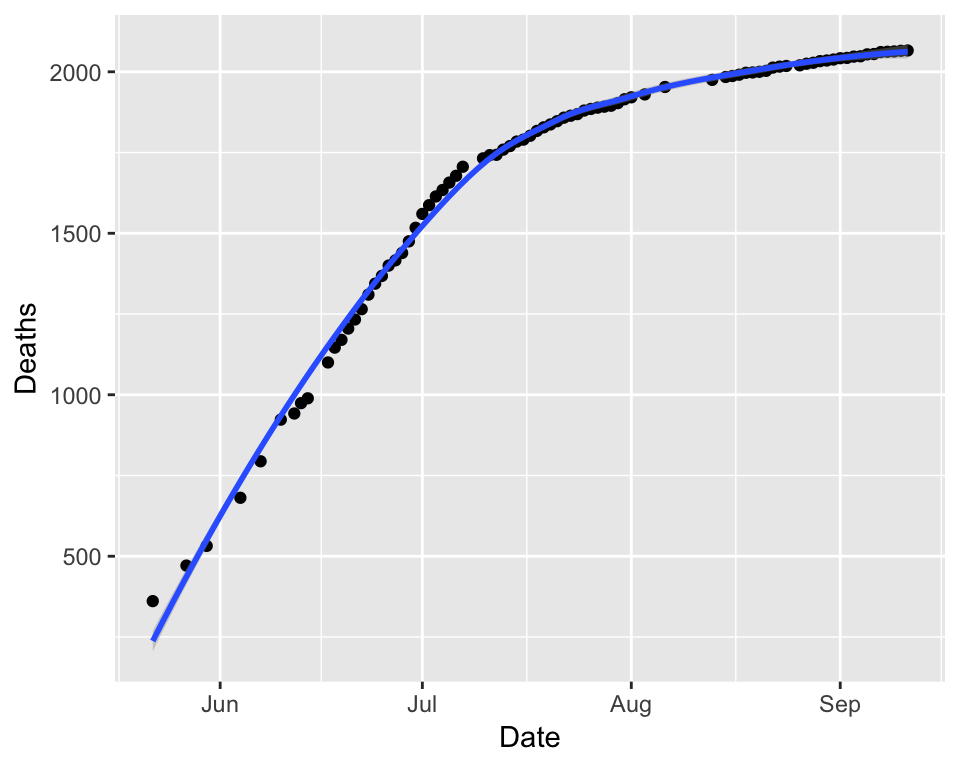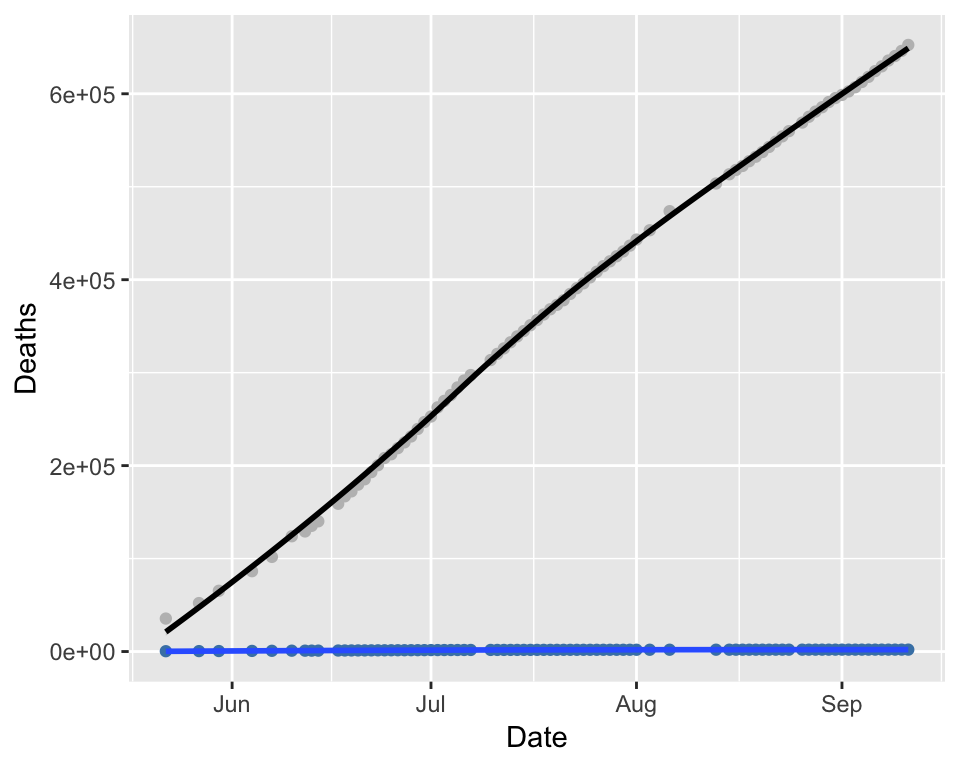### Load library & data set

``````library(ggplot2)

YemenData\$Date = as.Date(YemenData\$Date)#, format='%m/%d/%y')
YemenData = YemenData[order(YemenData\$Date),]
YemenData\$Times = as.numeric(YemenData\$Date-as.Date('5/22/2017', format='%m/%d/%Y'))``````

### Basic Plots

#### Simple Example

Let’s do a simple example:

``````# select the data  say which variables to use     draw as points
ggplot(YemenData,  aes(x=Date, y=Deaths))        + geom_point()``````You can also add some automatic processing, like a Loess-smoothed line:

``ggplot(YemenData, aes(x=Date, y=Deaths)) + geom_point() + geom_smooth(method = 'loess')``#### Slightly fancier plots

Let’s add some more variables and specify the colors! We can also make a variable to hold the plot (in this case `choleraplot`) so we can add things later on. If we make `choleraplot` a variable, then we’ll need to use `print(choleraplot)` to display the plot at the end.

``````choleraplot = ggplot(YemenData) +
geom_point(aes(x=Date, y=Deaths), color = 'steelblue') +
geom_smooth(aes(x=Date, y=Deaths), method = 'loess') +
geom_point(aes(x=Date, y=Cases), color = 'grey') +
geom_smooth(aes(x=Date, y=Cases), color = 'black', method = 'loess')
print(choleraplot)``````Looks nice, but the axis labels aren’t quite right, and we let’s add a title. We’ll do that with the `labs` function:

``````choleraplot = choleraplot + labs(title="Yemen Cholera Epidemic", x="Date", y="Number of Individuals")
print(choleraplot)``````